# Multivariate Spatial Regression Models¶

May 10, 2018 $\newcommand{\bs}{\boldsymbol}$ $\newcommand{\argmin}{\underset{\bs{#1}}{\text{arg min}}\,}$ $\newcommand{\argmax}{\underset{\bs{#1}}{\text{arg max}}\,}$ $\newcommand{\tr}{^{\top}}$ $\newcommand{\norm}{\left|\left|\,#1\,\right|\right|}$ $\newcommand{\given}{\,|\,}$ $\newcommand{\st}{\,\big|\,}$ $\newcommand{\E}{\mathbb{E}\left[#1\right]}$ $\newcommand{\P}{\mathbb{P}\left(#1\right)}$ $\newcommand{\abs}{\left|#1\right|}$ $\newcommand{\blue}{{\color{blue} {#1}}}$ $\newcommand{\red}{{\color{red} {#1}}}$ $\newcommand{\orange}{{\color{orange} {#1}}}$ $\newcommand{\pfrac}{\frac{\partial #1}{\partial #2}}$

A well designed spatial model is desirable when interpretability is more important than pure predictive power. It is the tradeoff between flexibility and interpretability. In this post I will touch the surface of multivariate spatial modeling, a vastly rich and exciting new field in statistical modeling.

If $s$ denotes a two-dimensional sites (usual consists of latitude and longitude), then we can interpret the univariate response $y(s)$ as a stochastic process. In addition, we assume that $Y(s)=(y_1(s),...,y_m(s))'$ is a $m\times 1$ response vector, such that

$$Y(s) = X\tr(s)\beta + W(s) + \epsilon(s). \tag{1}$$

Here the matrix $X\tr(s)$ is the $m\times p$ spatial covariate matrix formed as

$$X\tr(s) = \mathop{\oplus}_{k=1}^m x_k(s)\tr := \begin{bmatrix} x_1\tr(s) & \\ & \ddots & \\ & & x_m\tr(s) \end{bmatrix}, \tag{1'}$$

where $x_k(s)$ is the $p_k\times 1$ covariate vector for the $k$th component of $Y(s)$ and the notation $\oplus_{k=1}^m x_k\tr$ denotes a block diagonal matrix with $x_k\tr$ as its $k$th diagonal block.

The $p\times 1$ vector of regression coefficients

$$\beta = (\beta_1\tr,...,\beta_m\tr)'$$

is comprised by vertically stacking $m$ vectors of dimension $p_k \times 1$, where $\beta_k$ corresponds to $x_k\tr$, so that $p=\sum_{k=1}^m p_k$.

The most notable feature of the model is by assuming that the spatial process

$$W(s) =\big[W_i(s)\big]_{i=1}^m \sim \mathcal{G}_m(0, K(\cdot, \cdot)), \tag{2}$$

follows a $m\times 1$ zero-centered multivariate Gaussian process, which captures both the spatial dependence and the dependence among the $m$ components of $Y(s)$. The term $K(\cdot, \cdot)$ is the the $m\times m$ cross-covariance matrix function defined by

$$K(s, s')=\big[\text{cov}(W_i(s), W_j(s')) \big]_{i,j=1}^m,$$

which is a $m\times m$ matrix whose $(i,j)$th entry is the covariance function between Gaussian processess $W_i(s)$ and $W_j(s')$. Finally we assume that

$$\epsilon(s) \sim \mathcal{N}(0, \Psi),$$

where $\Psi$ is a $m\times m$ dispersion matrix and is independent of the spatial location $s$.

Given sites $s_1,...,s_n$, the we have a $mn \times 1$ response vector

$$Y = (Y\tr(s_1),...,Y\tr(s_n))',$$

where $Y(s_i)$ is a random vector of size $m\times 1$. The spatial random effect is a $mn\times 1$ multivariate normal distribution:

$$W=(W\tr(s_1),...,W\tr(s_n))' \sim \mathcal{N}(0, \Sigma_W),$$

where $\Sigma_W$ is the $mn\times mn$ cross-covariance matrix given by

$$\Sigma_W = \big[K(s_i, s_j)\big]_{i,j=1}^n,$$

a matrix comprising of $n\times n$ blocks of $m\times m$ cross-covariance matrices $K(s_i, s_j)$. From this setup, it follows that the response vector $Y$ follows a multivariate Gaussian distribution whose dispersion matrix is given by

$$\Sigma_Y = \Sigma_W + I_n \otimes \Psi,$$

where $I_n$ is the $n\times n$ identity matrix and $\otimes$ denotes the Kronecker product.

## Coregionalization Model¶

To ensure that the cross-covariance matrix $\Sigma_W$ is positive-definite, the cross-covariance matrix function needs to satisfy

$$K(s', s) = K\tr(s, s'). \tag{3}$$

The recent coregionalization model is developed constructively with computational feasibility in mind. We start with $m$ independent Gaussian processes with zero center and unit variance, which together forms a $m\times 1$ multivariate Gaussian process:

$$\tilde{W}(s)=\big[\tilde{W}_i(s)\big]_{i=1}^m,$$

where $\tilde{W}_k(s) \overset{ind.}{\sim} \mathcal{G}(0, \rho_k(\cdot, \cdot))$ are independently distributed univariate Gaussian processes with unit variance (i.e. $\text{var}(\tilde{W}_k(s_0))=1$ where $s_0$ is fixed) and the diagonal entries of the cross-covariance matrix function is

$$\tilde{K}(s, s')_{kk} = \text{cov}(\tilde{W}_k(s), \tilde{W}_k(s')) = \rho_k(s, s').$$

In the above formulation, the function $\rho_k(\cdot, \cdot)$ is a correlation function associated with the independent spatial proceess $\tilde{W}(s)$. By the unit variance assumption, we have that $\rho_k(s,s)=1$ for all $s$. It follows that $K(s, s')$ is a diagonal matrix function whose diagonal terms are $\rho_{k}(s,s')$ and the off-diagonal terms are all 0: i.e.,

$$\tilde{K}(s,s')_{kk'} = \text{cov}(\tilde{W}_k(s), \tilde{W}_{k'}(s')) = \begin{cases} \rho_k(s,s') & \text{ if } k=k' \\ 0 & \text{ if } k\neq k'. \end{cases}$$

It follows that $\tilde{K}(s,s')$ is a valid cross-covariance matrix function since it satisfies condition (3). There are many choices fo the correlation function $\rho(s,s')$. In this post we will use the Matérn correlation function given by

$$\rho(s,s'; \theta) = \frac{1}{2^{\nu-1}\Gamma(\nu)} \left(\norm{s-s'}\phi\right)^{\nu}\mathscr{K}_{\nu}\left(\norm{s-s'};\phi\right), \tag{4}$$

where $\theta := \{\phi, \nu\}$, $\phi>0$ is the spatial decay parameter, and $\nu>0$ is the smoothness parameter. Here $\Gamma{\cdot}$ is the usual Gamma function, and $\mathscr{K}_{\nu}$ is the Bessel function of the third kind with order $\nu$. The Matérn correlation function is isotropic since it only depends on the distance between sites.

To capture the covariance structure between the $m$ spatial processes $W_k(s)$, we take linear combinations of the independent spatial processes $\tilde{W}_k(s)$, so that the resulting multivariate processes is dependent. That is, we let

$$W(s)=A(s)\tilde{W}(s), \tag{5}$$

where $A(s)$ is a space-varying matrix-valued process that is invertible for all $s$.

Lemma 1   Under the transformation (5), the cross-covariance matrix function for $W_k(s)$ is related to $\tilde{K}_k(s)$ by

\begin{aligned} K(s,s') &= A(s)\tilde{K}(s,s')A\tr(s') \\ &= \sum_{k=1}^m a_k(s) a_k\tr(s')\rho_k(s,s'), \end{aligned} \tag{6}

where $a_k(s)$ is the $k$th column vector of $A(s)$, which can be found as the Cholesky decomposition of $\tilde{K}(s,s)$; i.e.,

$$A(s) = K^{1/2}(s,s). \tag{7}$$

Without loss of generality we assume that $A(s)$ is lower triangular. And $K(\cdot, \cdot)$ is a valid cross-covariance matrix function.

Proof   For sites $s$ and $s'$, the spatial random vector is a $2m\times 1$ vector that satisfies

$$\tilde{W} = \begin{bmatrix}\tilde{W}(s_1) \\ \tilde{W}(s_2)\end{bmatrix} \sim \mathcal{N}\left\{0,\, \Sigma_{\tilde{W}} \right\},$$

where the cross-covariance matrix is given by

$$\Sigma_{\tilde{W}} = \begin{bmatrix} \tilde{K}(s_1,s_1) & \tilde{K}(s_1,s_2) \\ \tilde{K}(s_2, s_1) & \tilde{K}(s_2,s_2) \end{bmatrix} = \begin{bmatrix} I_m & \otimes_{k=1}^m \rho_k(s_1, s_2) \\ \otimes_{k=1}^m \rho_k(s_2, s_1) & I_m \end{bmatrix}. \tag{8}$$

After applying the linear map (5), the transformed spatial random effect is

$$W = \begin{bmatrix}A(s_1)\tilde{W}(s_1) \\ A(s_2)\tilde{W}(s_2)\end{bmatrix} = \begin{bmatrix} A(s_1) & 0 \\ 0 & A(s_2) \end{bmatrix} \tilde{W} := \mathscr{A} \tilde{W}.$$

Thus, the transformed cross-covariance matrix has the following distribution:

\begin{aligned} W &\sim \mathcal{N}\left\{0, \Sigma_W\right\} \\ &= \mathcal{N}\left\{0, \mathscr{A}\Sigma_{\tilde{W}}\mathscr{A}\tr\right\} \\ &= \mathcal{N}\left\{0, \,\, \begin{bmatrix} A(s_1)A\tr(s_1) & A(s_1)\tilde{K}(s_1,s_2)A\tr(s_2) \\ A(s_2)\tilde{K}(s_2,s_1)A\tr(s_1) & A(s_2) A\tr(s_2) \end{bmatrix}\right\}. \end{aligned}

Since $\tilde{K}(s_i, s_j)$ is a diagonal matrix, relations (6) and (7) are proved. The positive-definiteness of the cross-covariance matrix $\Sigma_W$ also follows. $\blacksquare$

The proof of Lemma 1 can be easily generalized. Suppose we have $n$ sites $s_1,...,s_n$. The spatial random vector becomes

$$W = \big[W(s_i)\big]_{i=1}^n \sim \mathcal{N}(0, \Sigma_W),$$

where the cross-covariance matrix is given by

\begin{aligned} \Sigma_W &= \big[\mathop{\oplus}_{i=1}^n A(s_i)\big] \big[\mathop{\oplus}_{k=1}^m \rho_k(s_i, s_j) \big]_{i,j=1}^n \big[\mathop{\oplus}_{i=1}^n A\tr(s_i) \big] \\ &:= \mathscr{A} \Sigma_{\tilde{W}}\mathscr{A}\tr. \end{aligned} \tag{8'}

For simplication, as the Matérn correlation function is isotropic, we define

$$\mathcal{K}(s-s') := K(s,s'),$$

which only depends on the separation between sites. As a result, we have that $K(s,s)=\mathcal{K}(0)$ so that

$$A:= A(s) = \mathcal{K}^{1/2}(0). \tag{7'}$$

In this case we have $\mathscr{A}= I_n \otimes A$, and $(8')$ reduces to

$$\Sigma_W = (I_n \otimes A)\Sigma_{\tilde{W}} (I_n\otimes A\tr).$$

For a further simplication, we could assume the same correlation function for each component of $\tilde{W}(s)$; that is,

$$\tilde{K}(s,s') = \rho(s,s')I_m.$$

The original cross-covariance matrix (8) reduces to

$$\Sigma_{\tilde{W}} = R \otimes I_m, \quad R := \big[\rho(s_i, s_j) \big]_{i,j=1}^n, \tag{9}$$

and the transformed cross-covariance matrix (8') becomes

$$\Sigma_W = (I_n \otimes A) (R\otimes I_m) (I_n \otimes A\tr) = R \otimes \mathcal{K}(0). \tag{9'}$$

Equations (9) and (10) are referred to as separable specifications of the covariance structure since the cross-covariance matrix is separated into the spatial component $R$ and a within-site dispersion matrix $\mathcal{K}(0)$. Such model is easy to fit and have nice intepretability but poor flexibility.

## Full Model Specification¶

The model follows a generic template:

$$Y=X\beta + \mathscr{A}\tilde{W} + \epsilon; \quad \epsilon \sim \mathcal{N}(0, I_m\otimes \Psi). \tag{10}$$

In this model, the response $Y$ is a $mn\times 1$ vector, and $X$ is a $mn\times p$ design matrix formed by stacking the spatial covariate matrices defined in (1'):

$$X = \big[X\tr(s_i) \big]_{i=1}^n.$$

To reduce the parameter space, we integrate out $\tilde{W}$ to obtain the marginalized likelihood:

$$Y \given \Omega \sim \mathcal{N}(X\beta, \mathscr{A}\Sigma_{\tilde{W}}\mathscr{A}\tr + I_n\otimes \Psi),$$

where $\Omega = (\beta, \mathscr{A}, \theta, \Psi)$ is the set of parameters that are to be estimated in the MCMC procedure.

\begin{aligned} \beta &\sim \mathcal{N}(\mu_\beta, \Sigma_\beta) \\ \Psi &\sim \otimes_{i=1}^m \tau_i^2 \\ \tau_i^2 &\sim \text{IG}(a_i, b_i). \end{aligned}

The matrix $\mathscr{A}$ depends on the exact form of $A$, which is a lower triangular matrix defined in (7') to represent the within-site dispersion structure. Using an isotropic correlation function, we have that $\mathscr{A}=I_n\otimes A$, and an inverse-Wishart prior is specified for $AA\tr$:

$$\mathcal{K} := A A\tr \sim \text{IW}(w, \Phi).$$

Finally, we assign priors to the parameters of the Matén correlation $\theta:=\{\phi_k, \nu_k\}_{k=1}^m$, which are usually taken to be uniform. Motivated by earlier research, the decay parameters $\phi_k$ are typically set to reflect the relative size of the spatial domain, and the smoothness parameters $\nu_k$ are often estimated using a uniform distribution. This results in a final posterior distribution:

$$p(\Omega \given \text{Data}) \propto p(Y\given \beta, \mathscr{A}, \theta, \Psi)p(\beta) p(\mathscr{A})p(\theta)p(\Psi). \tag{11}$$

The parameter $\beta$ can be updated using Gibbs sampling from $\mathcal{N}(\mu_{\beta_{new}}, \Sigma_{\beta_{new}})$, where

\begin{aligned} \Sigma_{\beta_{new}} &= \big[\Sigma_{\beta}^{-1} + X\tr(\mathscr{A}\Sigma_{\tilde{W}}\mathscr{A}\tr + I_n\otimes \Psi)^{-1}X\big]^{-1} \\ \mu_{\beta_{new}} &= \Sigma_{\beta_{new}} X\tr(\mathscr{A}\Sigma_{\tilde{W}}\mathscr{A}\tr + I_n\otimes \Psi)^{-1}Y. \end{aligned}

The remaining parameters must be updated using the Metropolis-Hastings algorithm.

## Posterior Recovery¶

We did not sample $\tilde{W}$ because we want a smaller parameter space for a more efficient MCMC algorithm. Nevertheless, the posterior distribution of $\tilde{W}$ can be recovered using a compoisition sampling:

$$p(\tilde{W}\given \text{Data}) \propto \int p(\tilde{W}\given \Omega, \text{Data}) p(\Omega \given \text{Data})\, d\Omega. \tag{12}$$

The MCMC produces a set of parameters $\{\Omega^{(k)}\}_{k=1}^N$, which forms the empirical distribution for $p(\Omega \given \text{Data})$. We can randomly draw a realization $\Omega^{(k)}$ from $p(\Omega \given \text{Data})$, and use it to sample $\tilde{W}^{(k)}$ from $p(\tilde{W}\given \Omega^{(k)}, \text{Data})$, which can be found as follows.

From (10) by letting $Y^* = Y-X\beta$, we have

$$Y^* \sim \mathcal{N}(\mathscr{A}\tilde{W}, I_n \otimes \Psi).$$

Letting $\mathscr{E} = (I_n\otimes \Psi)^{-1}=I_n\otimes \Psi^{-1}$, and adding the prior $\tilde{W}\sim \mathcal{N}(0, \Sigma_{\tilde{W}})$, we have

\begin{aligned} p(\tilde{W} \given \Omega, \text{Data}) &\propto \text{exp}\left\{-\frac{1}{2}\left((Y^*-\mathscr{A}\tilde{W})\tr\mathscr{E}(Y^*-\mathscr{A}\tilde{W}) + \tilde{W}\tr\Sigma_{\tilde{W}}^{-1}\tilde{W}\right) \right\} \\ &\propto \text{exp}\left\{-\frac{1}{2}\left(\tilde{W}\tr \left[\Sigma_{\tilde{W}}^{-1} + \mathscr{A}\tr\mathscr{E}\mathscr{A}\right] \tilde{W} - 2{Y^*}\tr\mathscr{E}\mathscr{A}\tilde{W}\right) \right\} \end{aligned}

The kernel of the multivariate Gaussian $W\sim \mathcal{N}(\mu, \Sigma)$ is

$$p(W) \propto \text{exp}\left\{-\frac{1}{2}\left(W\tr \Sigma^{-1}W-2\mu\tr \Sigma^{-1}W\right) \right\},$$

which is used as a comparison to conclude that

$$p(\tilde{W}\given \Omega, \text{Data}) \sim \mathcal{N}(\mu_{\tilde{W}_{new}}, \Sigma_{\tilde{W}_{new}}),$$

where the posterior mean and variance is given by

\begin{aligned} \Sigma_{\tilde{W}_{new}} &= \left(\Sigma_{\tilde{W}}^{-1} + \mathscr{A}\tr(I_n\otimes \Psi^{-1})\mathscr{A}\right)^{-1} \\ \mu_{\tilde{W}_{new}} &= \Sigma_{\tilde{W}_{new}}\mathscr{A}\tr(I_n\otimes \Psi^{-1})(Y-X\beta). \end{aligned}

## Posterior Prediction¶

Now we are interested in prediction at new sites. Let $\{s^*_k\}_{k=1}^{n^*}$ be a collection of $n^*$ new sites, and define $\tilde{W}^* := \big[\tilde{W}(s^*_k)\big]_{k=1}^{n^*}$ be the spatial random vector for the new sites. Its distribution can be found the same way as (12) using compoisition sampling:

$$p(\tilde{W}^*\given \text{Data}) \propto \int p(\tilde{W}^* \given \tilde{W}, \Omega, \text{Data})p(\tilde{W}\given \Omega, \text{Data})p(\Omega\given \text{Data})\,d\Omega\,d\tilde{W}.$$

The final step of the compoisition sampling requires the posterior distirbution $p(\tilde{W}^* \given \cdot)$, which can be found as follows. First we stack the seen and unseen random effect vectors in a new vector:

$$\begin{bmatrix} \tilde{W}\\ \tilde{W}^*\end{bmatrix} \sim \mathcal{N}\left\{\begin{bmatrix} 0 \\ 0 \end{bmatrix}, \quad \begin{bmatrix} \Sigma_{\tilde{W}} & \Sigma_{\tilde{W}, \tilde{W}^*} \\ \Sigma_{\tilde{W}^*, \tilde{W}} & \Sigma_{\tilde{W}^*} \end{bmatrix}\right\}$$

where the combined covariance structure is given by

\begin{aligned} \Sigma_{\tilde{W}} &= \left[\mathop{\oplus}_{k=1}^m \rho_k(s_i, s_j) \right]_{i,j=1}^n \\ \Sigma_{\tilde{W}^*} &= \left[\mathop{\oplus}_{k=1}^m \rho_k(s_i^*, s_j) \right]_{i,j=1}^{n^*} \\ \Sigma_{\tilde{W}^*,\tilde{W}} &= \Sigma\tr_{\tilde{W},\tilde{W}^*} = \left[\mathop{\oplus}_{k=1}^m\rho_k(s_i^*, s_j) \right]_{i=1,j=1}^{i=n^*,j=n}. \end{aligned}

The distribution $p(\tilde{W}^*\given \tilde{W}, \Omega, \text{Data})$ is the conditional distribution of multivariate normal $\mathcal{N}(\mu_{\tilde{W}^*\given\tilde{W}}, \Sigma_{\tilde{W}^*\given\tilde{W}})$, where

\begin{aligned} \mu_{\tilde{W}^*\given\tilde{W}} &= \Sigma_{\tilde{W}^*, \tilde{W}}\Sigma_{\tilde{W}}^{-1} \tilde{W} \\ \Sigma_{\tilde{W}^*\given\tilde{W}} &= \Sigma_{\tilde{W}^*} - \Sigma_{\tilde{W}^*,\tilde{W}}\Sigma_{\tilde{W}}^{-1}\Sigma_{\tilde{W},\tilde{W}^*}. \end{aligned}

After obtaining samples $\{\tilde{W}^{*(k)}\}_{k=1}^N$, the corresponding conditional expectation of the response $Y^* = \left[Y(s_i)^*\right]_{i=1}^{n^*}$ can be sampled as

$$E\left[Y^* \given \text{Data}\right]^{(k)} = X^*\beta^{(k)} + \mathscr{A}^{(k)}\tilde{W}^{*(k)},$$

for $k=1,...,N$ and where $X^*$ is the $mn^*\times p$ design matrix for the new sites.

Alternatively, if prediction the primary goal, $\tilde{W}^*$ does not need to be sampled directly. Instead, the $Y^*$ can be sample via the composite sampling:

$$p(Y^*\given \text{Data}) \propto \int p(Y^* \given \Omega, \text{Data})p(\Omega\given \text{Data})\, d\Omega.$$

The final distribution $p(Y*\given \Omega, \text{Data})$ can be found similarly as the conditional distribution of multivariate normal:

$$\begin{bmatrix} Y\\ Y^*\end{bmatrix} \sim \mathcal{N}\left\{\begin{bmatrix} X\beta \\ X^*\beta \end{bmatrix}, \quad \begin{bmatrix} \Sigma_{Y} & \Sigma_{Y,Y^*} \\ \Sigma_{Y^*,Y} & \Sigma_{Y^*} \end{bmatrix}\right\},$$

where the conbined covariance structure is given by

\begin{aligned} \Sigma_{Y} &= \mathscr{A}\Sigma_{\tilde{W}}\mathscr{A}\tr + I_n\otimes\Psi, \text{ where } \mathscr{A}=I_n\otimes A \\ \Sigma_{Y^*} &= \mathscr{A}^*\Sigma_{\tilde{W}^*}{\mathscr{A}^{*}}\tr, \text{ where } \mathscr{A}=I_{n^*}\otimes A \\ \Sigma_{Y^*, Y} &= \mathscr{A}^*\Sigma_{\tilde{W}^*,\tilde{W}}\mathscr{A}\tr. \end{aligned}

From this we conclude that $Y^*\given \Omega, \text{Data} \sim \mathcal{N}(\mu_{Y^*\given Y}, \Sigma_{Y^*\given Y})$, where

\begin{aligned} \mu_{Y^*\given Y} &= X^*\beta + \Sigma_{Y^*,Y}\Sigma_Y^{-1}(Y-X\beta), \\ \Sigma_{Y^*\given Y} &= \Sigma_{Y^*} - \Sigma_{Y^*, Y} \Sigma_Y^{-1}\Sigma_{Y,Y^*}. \end{aligned}

## Implementation using spBayes¶

The model can be fit using the spBayes package.

In :
require(ggplot2)
require(spBayes)
require(ggpubr)


First we propose an efficient way to sample from a multivariate normal distribution. First we generate a $p$-dimensional random vector $z\sim \mathcal{N}(0, I_p)$. This can be done easily by generating $p$ individual standard normal random variables. The transformed variable $Dz$, where $D$ is a $p\times p$ matrix, follows a $\mathcal{N}(0, V)$ distribution where $V=DD\tr$, so that $D$ can be taken as the Cholesky decomposition of $V$. This motivates the following method.

In :
# sampling from multivariate normal
rmvn <- function(n, mu=0, V=matrix(1)){
p <- length(mu)
if(any(is.na(match(dim(V),p)))){stop("Dimension problem!")}
D <- chol(V)
t(matrix(rnorm(n*p), ncol=p)%*%D + rep(mu, rep(n,p)))
}


Now we generate our data according to the general template (10), with the separable specification in the covariance structure as in (9) and (9'). That is,

$$\mathscr{A}=I_n\otimes A, \quad \Sigma_{\tilde{W}} = \left[\tilde{K}(s_i, s_j)\right]_{i,j=1}^n,$$

and $\tilde{K}(s_i, s_j) = \otimes_{1}^m \rho(s_i, s_j; \theta)$, where $\rho$ is the isotropic Matérn correlation function, and $\theta=(\phi, \nu)$ represent its decay and smoothness. We take $A$ to be the lower triangular matrix

$$A = \begin{bmatrix} -1 & 0 \\ 2 & 1 \end{bmatrix}, \quad \mathcal{K} = AA\tr = \begin{bmatrix} 1 & -2 \\ -2 & 5 \end{bmatrix}.$$

In :
data.make <- function(seed=1, n=50, m=2, phi=c(6,6), nu=c(2,2), A.lower = c(-1,2,1),
B.1=1, B.2=1, Psi=diag(c(1,1))){
#     -----------
#     n = number of sites
#     m = dimension of the response vector Y
#     phi = decay parameter for Matern (dim = m)
#     nu = smoothness parameter for Matern (dim = m)
#     A.lower = lower triangular matrix for within-site covariance K
#     B.1, B.2 = coefficients for the constant fixed effect
#     Psi = covariance matrix for the error
#     -----------
if(any(is.na(match(c(length(phi), length(nu)), m)))){stop("Dimension problem!")}
set.seed(seed)
coords <- cbind(runif(n,0,1), runif(n,0,1)) # generate locations
nltr <- m*(m+1)/2 # number of triangular elements in the cross-covariance matrix
if(is.na(match(length(A.lower), nltr))){stop("Dimension problem!")}
theta <- c(phi, nu) # spatial decay (phi) + spatial smoothness (nu)
A <- matrix(0,m,m) # creating lower triangular A
A[lower.tri(A,TRUE)] <- A.lower # creating lower triangular A
K <- A%*%t(A) # within-site covariance matrix K = AA^t
Psi.C <- diag(0,m) # dispersion matrix for the random error
C <- mkSpCov(coords, K, Psi.C, theta, cov.model='matern') # spatial covariance matrix
w <- rmvn(1, rep(0, nrow(C)),C) # spatial random effects
w.1 <- w[seq(1,length(w),m)] # spatial random effect for component 1
w.2 <- w[seq(2,length(w),m)] # spatial random effect for component 2
x.1 <- matrix(rep(1,n),nrow=n) # design matrix for component 1
x.2 <- matrix(rep(1,n),nrow=n) # design matrix for component 2
x <- mkMvX(list(x.1, x.2)) # multivariate design matrix
B <- c(B.1,B.2) # fixed effect
y <- rnorm(n*m, x%*%B+w, diag(n)%x%Psi) # outcome vector
list('location'=coords, 'w'=w, 'w1'=w.1, 'w2'=w.2, 'y'=y, 'x'=x)
}


Since the response vector $Y$ is binary, we can visualize the spatial data as a vector field. In the following grid plot, we examine the effect of the decay parameter $\phi$ and smoothness parameter $\nu$ on the distribution of the bivariate response variable using $n=30$ sites.

In :
data.plot <- function(y, coords, phi=3, nu=2, n=30, m=2){
label <- matrix(y, byrow=TRUE, ncol=m) # stack response Y into a matrix
df <- data.frame(cbind(coords, label))
colnames(df) <- c('lat', 'lon', 'y1', 'y2')
v.len <- mean(sqrt(df$y1^2+df$y2^2)) # scaling vector lengths
p <- {ggplot(data=df, aes(x=lat, y=lon)) +
geom_point(size=.5, col='red') +
geom_segment(aes(xend=lat+y1/(10*v.len), yend=lon+y2/(10*v.len)),
arrow = arrow(length = unit(0.1,"cm")), col='red') +
ggtitle(bquote(phi==.(phi)~',' ~nu==.(nu))) +
theme(axis.title.x = element_blank(), axis.title.y = element_blank())}
return(p)
}

In :
params <- expand.grid(phi = c(1,3,5), nu = c(0.5,1,2))
p <- list()

for (i in 1:nrow(params)){
phi <- unlist(params[i,])
nu <- unlist(params[i,])
data <- data.make(seed=3, n=30, m=2, phi=rep(phi,2), nu=rep(nu,2),
A.lower = c(-1,2,1), B.1=1, B.2=1, Psi=diag(c(1,1)))
coords <- data$location y <- data$y
p[[i]] <- data.plot(y, coords, phi, nu)
}

In :
options(repr.plot.width=8.5, repr.plot.height=8.5)
ggarrange(p[], p[], p[],
p[], p[], p[],
p[], p[], p[], nrow=3, ncol=3)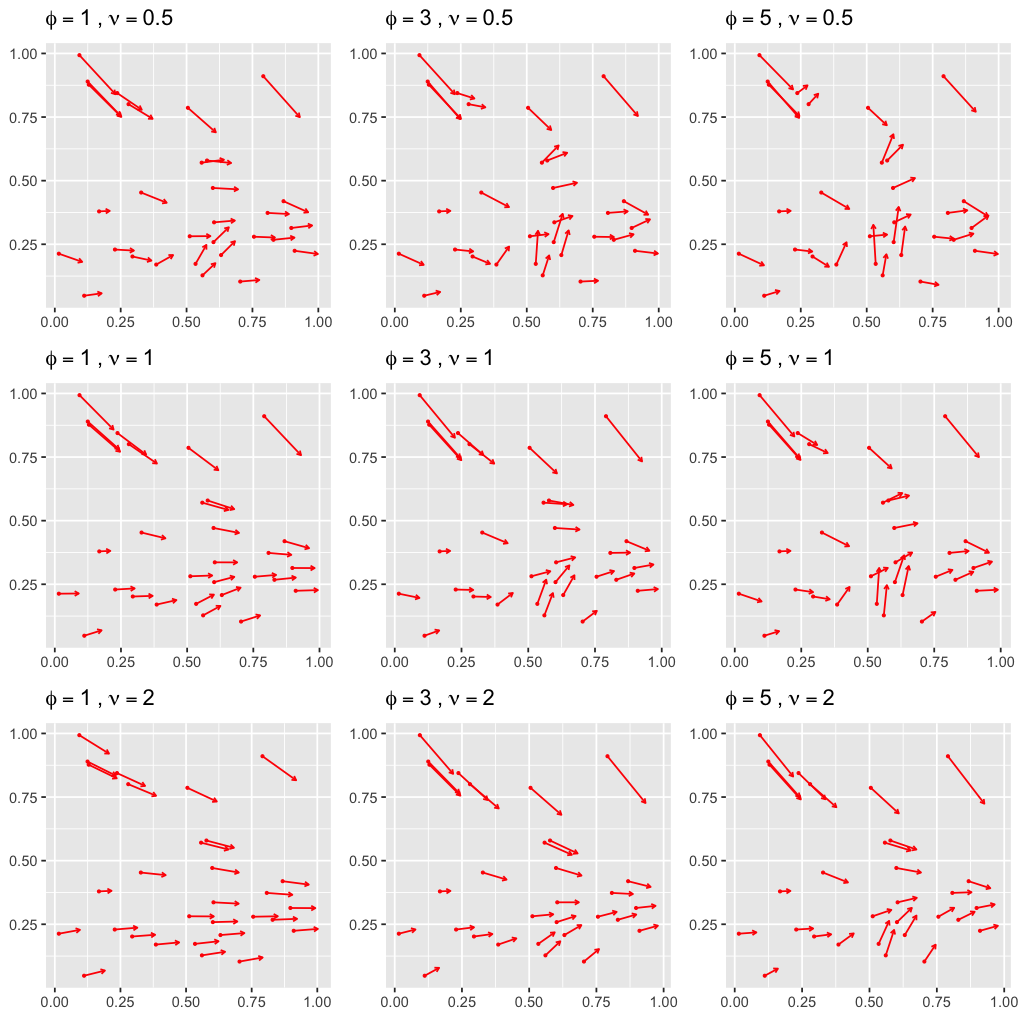For our analysis we will use $n=50$, $\phi=5$ and $\nu=1$. This is plotted below.

In :
data <- data.make(seed=3, n=50, m=2, phi=rep(5,2), nu=rep(1,2),
A.lower = c(-1,2,1), B.1=1, B.2=1, Psi=diag(c(1,1)))
coords <- data$location y <- data$y

options(repr.plot.width=4, repr.plot.height=4)
print(data.plot(y, coords, phi=5, nu=1))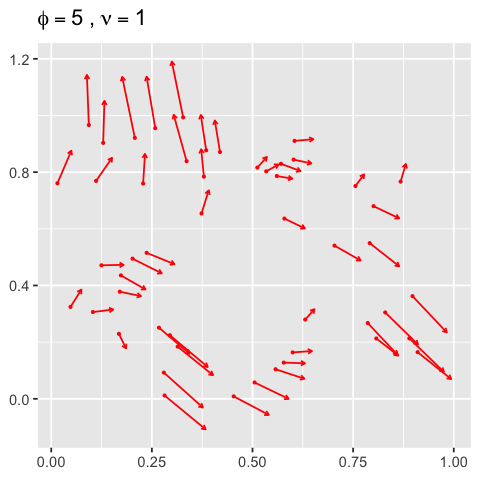Now we are ready to load the various components for fitting the model.

In :
m <- 2; n <- 50
y.1 <- y[seq(1,length(y),m)] # response for first component
y.2 <- y[seq(2,length(y),m)] # response for second component

# spatial random effects
w <- data$w w.1 <- data$w1
w.2 <- data$w2 # Covariate portion of the mean x.1 <- matrix(rep(1,n), n, 1) x.2 <- matrix(rep(1,n), n, 1) # create a multivariate design matrix given q univariate design matrices x <- mkMvX(list(x.1, x.2)) # number of MCMC iterations for spMvLM() n.samples <- 10000 # starting values for the MCMC A.starting <- diag(2,m)[lower.tri(diag(2,m), TRUE)] starting <- list("phi"=rep(5,m), "nu"=rep(1,m), "A"=A.starting, "Psi"=rep(1,m)) # Metropolis proposal sampler variances tuning <- list("phi"=rep(0.6,m), "nu"=rep(0.35,m), "A"=rep(0.03,length(A.starting)), "Psi"=rep(0.008,m)) # prior values priors <- list("beta.Flat", "phi.Unif"=list(rep(4,m), rep(6,m)), "nu.Unif"=list(rep(0,m), rep(2,m)), "K.IW"=list(m+1, diag(0.5,m)), "Psi.ig"=list(c(2,2), c(.1,.1))) # fitting the model using spMvLM() function m.1 <- spMvLM(list(y.1~x.1-1, y.2~x.2-1), coords=coords, starting=starting, tuning=tuning, priors=priors, n.samples=n.samples, cov.model="matern", n.report=2500)  ---------------------------------------- General model description ---------------------------------------- Model fit with 50 observations. Number of covariates 2 (including intercept if specified). Using the matern spatial correlation model. Number of MCMC samples 10000. Priors and hyperpriors: beta flat. K IW hyperpriors df=3.00000, S= 0.500 0.000 0.000 0.500 Diag(Psi) IG hyperpriors parameter shape scale Psi[1,1] 2.0 0.10 Psi[2,2] 2.0 0.10 phi Unif hyperpriors parameter a b phi 4.00000 6.00000 phi 4.00000 6.00000 nu Unif hyperpriors nu 0.00000 2.00000 nu 0.00000 2.00000 ------------------------------------------------- Sampling ------------------------------------------------- Sampled: 2500 of 10000, 25.00% Report interval Metrop. Acceptance rate: 20.44% Overall Metrop. Acceptance rate: 20.44% ------------------------------------------------- Sampled: 5000 of 10000, 50.00% Report interval Metrop. Acceptance rate: 17.56% Overall Metrop. Acceptance rate: 19.00% ------------------------------------------------- Sampled: 7500 of 10000, 75.00% Report interval Metrop. Acceptance rate: 16.68% Overall Metrop. Acceptance rate: 18.23% ------------------------------------------------- Sampled: 10000 of 10000, 100.00% Report interval Metrop. Acceptance rate: 14.36% Overall Metrop. Acceptance rate: 17.26% -------------------------------------------------  In : burn.in <- 0.5*n.samples options(repr.plot.width=8, repr.plot.height=8) par(mfrow=c(3,3)) ts.plot(m.1$p.theta.samples[burn.in:n.samples,1],ylab="",main="K[1,1]")
ts.plot(m.1$p.theta.samples[burn.in:n.samples,2],ylab="",main="K[2,1]") ts.plot(m.1$p.theta.samples[burn.in:n.samples,3],ylab="",main="K[2,2]")
ts.plot(m.1$p.theta.samples[burn.in:n.samples,4],ylab="",main="Psi[1,1]",ylim=c(0,.1)) ts.plot(m.1$p.theta.samples[burn.in:n.samples,5],ylab="",main="Psi[2,2]",ylim=c(0.005,.1))
ts.plot(m.1$p.theta.samples[burn.in:n.samples,6],ylab="",main="phi") ts.plot(m.1$p.theta.samples[burn.in:n.samples,7],ylab="",main="phi")
ts.plot(m.1$p.theta.samples[burn.in:n.samples,8],ylab="",main="nu") ts.plot(m.1$p.theta.samples[burn.in:n.samples,9],ylab="",main="nu")In :
m.1 <- spRecover(m.1, start=burn.in, thin=5)

-------------------------------------------------
Recovering beta and w
-------------------------------------------------
Sampled: 99 of 1001, 9.89%
Sampled: 199 of 1001, 19.88%
Sampled: 299 of 1001, 29.87%
Sampled: 399 of 1001, 39.86%
Sampled: 499 of 1001, 49.85%
Sampled: 599 of 1001, 59.84%
Sampled: 699 of 1001, 69.83%
Sampled: 799 of 1001, 79.82%
Sampled: 899 of 1001, 89.81%
Sampled: 999 of 1001, 99.80%

In :
# quantiles for theta and beta parameters
round(summary(m.1$p.theta.recover.samples)$quantiles[,c(3,1,5)],2)
round(summary(m.1$p.beta.recover.samples)$quantiles[,c(3,1,5)],2)

50%2.5%97.5%
K[1,1] 0.79 0.40 1.75
K[2,1]-1.69-3.54-0.85
K[2,2] 4.65 2.51 8.58
Psi[1,1] 0.03 0.01 0.06
Psi[2,2] 0.04 0.02 0.08
phi 5.11 4.06 5.96
phi 4.83 4.04 5.80
nu 1.02 0.69 1.43
nu 1.43 0.73 1.91
50%2.5%97.5%
x.1.mod10.98 0.061.86
x.2.mod20.73 -1.303.00
In :
# quantiles for spatial random effects
w.hat <- summary(mcmc(t(m.1$p.w.recover.samples)))$quantiles[,c(3,1,5)]
w.1.hat <- w.hat[seq(1, nrow(w.hat), m),]
w.2.hat <- w.hat[seq(2, nrow(w.hat), m),]

options(repr.plot.width=7, repr.plot.height=4)
par(mfrow=c(1,2))
plot(w.1, w.1.hat[,1], xlab="Real", ylab="Fitted",
xlim=range(w.1), ylim=range(w.1.hat), main="w.1")
arrows(w.1, w.1.hat[,1], w.1, w.1.hat[,2], length=0.02, angle=90, col='lightblue')
arrows(w.1, w.1.hat[,1], w.1, w.1.hat[,3], length=0.02, angle=90, col='lightblue')
lines(range(w), range(w), col='red')

# plot for w.2 spatial random effects
plot(w.2, w.2.hat[,1], xlab="Real", ylab="Fitted",
xlim=range(w.2), ylim=range(w.2.hat), main="w.2")
arrows(w.2, w.2.hat[,1], w.2, w.2.hat[,2], length=0.02, angle=90, col='lightblue')
arrows(w.2, w.2.hat[,1], w.2, w.2.hat[,3], length=0.02, angle=90, col='lightblue')
lines(range(w), range(w), col='red')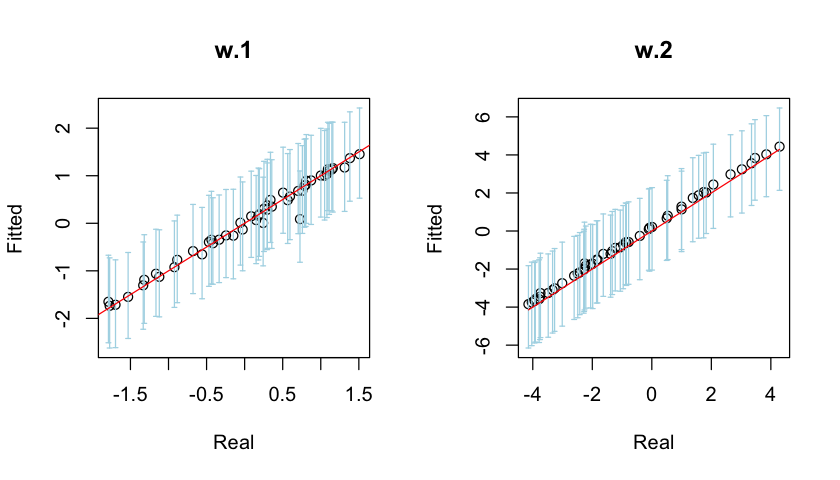The two Gaussian processes $W_1(s)$ and $W_2(s)$ can be visualized by their interpolated surfaces of the posterior mode. This can be achieved using the MBA and the fields packages.

In :
require(MBA)
require(fields)
xyz.1 <- as.matrix(cbind(coords, w.1.hat[,1]))
xyz.2 <- as.matrix(cbind(coords, w.2.hat[,1]))
xyz.3 <- as.matrix(cbind(coords, w.1))
xyz.4 <- as.matrix(cbind(coords, w.2))
mba.int.1 <- mba.surf(xyz.1, 300, 300, extend=TRUE)$xyz.est mba.int.2 <- mba.surf(xyz.2, 300, 300, extend=TRUE)$xyz.est
mba.int.3 <- mba.surf(xyz.3, 300, 300, extend=TRUE)$xyz.est mba.int.4 <- mba.surf(xyz.4, 300, 300, extend=TRUE)$xyz.est

options(repr.plot.width=7, repr.plot.height=7)
par(mfrow=c(2,2))
image.plot(mba.int.1, xaxs="r", yaxs="r", main='Fitted W.1')
image.plot(mba.int.2, xaxs="r", yaxs="r", main='Fitted W.2')
image.plot(mba.int.3, xaxs="r", yaxs="r", main="Real W.1")
image.plot(mba.int.4, xaxs="r", yaxs="r", main='Real W.2')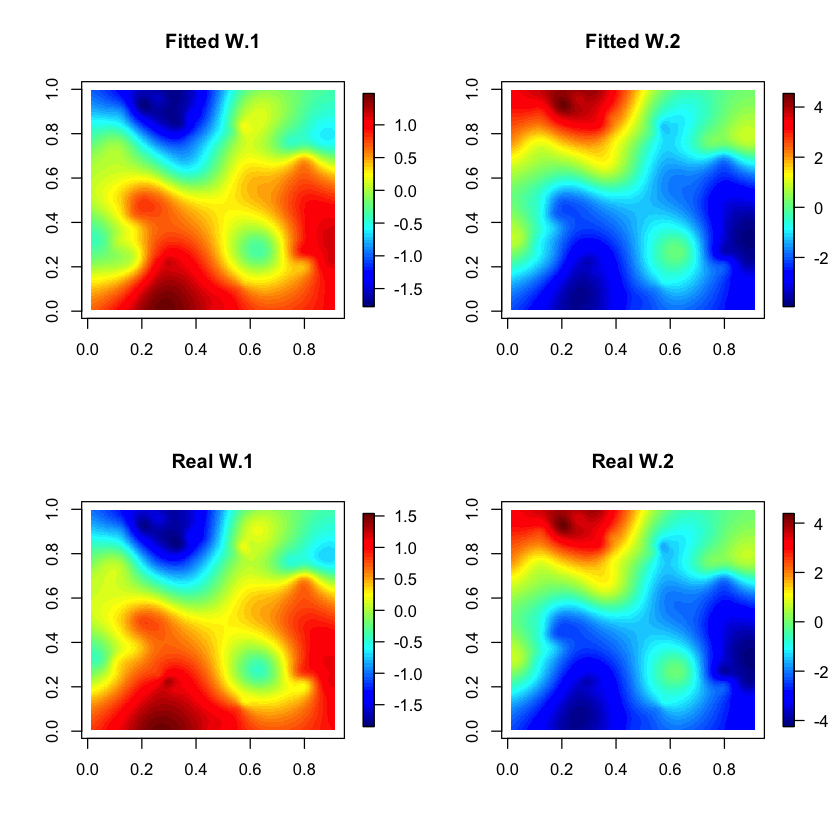Posterior prediction can be done using the spPredict function. We make our prediction on a $9\times 9$ grid on the unit square $[0,1]^2$. Note that $n^*=9\times 9 = 81$.

In :
# new coordinates of dimension (81x2)
l <- seq(.1,.9,.1)
coords.new <- as.matrix(expand.grid(l,l))

# new design matrix
x.1.new <- matrix(rep(1,81),nrow=81) # design matrix for component 1
x.2.new <- matrix(rep(1,81),nrow=81) # design matrix for component 2
x.new <- mkMvX(list(x.1.new, x.2.new)) # multivariate design matrix

# posterior prediction
m.1.pred <- spPredict(m.1, start = burn.in, thin = 5, pred.covars = x.new, pred.coords = coords.new)

-------------------------------------------------
Recovering beta
-------------------------------------------------
Sampled: 99 of 1001, 9.89%
Sampled: 199 of 1001, 19.88%
Sampled: 299 of 1001, 29.87%
Sampled: 399 of 1001, 39.86%
Sampled: 499 of 1001, 49.85%
Sampled: 599 of 1001, 59.84%
Sampled: 699 of 1001, 69.83%
Sampled: 799 of 1001, 79.82%
Sampled: 899 of 1001, 89.81%
Sampled: 999 of 1001, 99.80%
----------------------------------------
General model description
----------------------------------------
Model fit with 50 observations.

Prediction at 81 locations.

Number of covariates 2 (including intercept if specified).

Using the matern spatial correlation model.

-------------------------------------------------
Sampling
-------------------------------------------------
Sampled: 100 of 1001, 9.89%
Sampled: 200 of 1001, 19.88%
Sampled: 300 of 1001, 29.87%
Sampled: 400 of 1001, 39.86%
Sampled: 500 of 1001, 49.85%
Sampled: 600 of 1001, 59.84%
Sampled: 700 of 1001, 69.83%
Sampled: 800 of 1001, 79.82%
Sampled: 900 of 1001, 89.81%
Sampled: 1000 of 1001, 99.80%

In :
# obtain prediction using posterior mean
y.new <- apply(m.1.pred$p.y.predictive.samples, 1, mean) # plotting prediction options(repr.plot.width=4, repr.plot.height=4) print(data.plot(y.new, coords.new, phi=5, nu=1))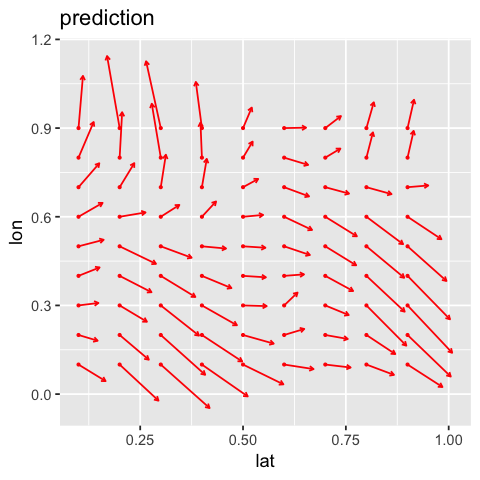Finally we compare the interpolated surfaces generated using both the original response y and the fitted response y.new. A slight overestimation in the first component is observed. Otherwise, the fit is very good. In : y.1.new <- y.new[seq(1, length(y.new), 2)] y.2.new <- y.new[seq(2, length(y.new), 2)] xyz.1 <- as.matrix(cbind(coords.new, y.1.new)) xyz.2 <- as.matrix(cbind(coords.new, y.2.new)) xyz.3 <- as.matrix(cbind(coords, y.1)) xyz.4 <- as.matrix(cbind(coords, y.2)) mba.int.1 <- mba.surf(xyz.1, 300, 300, extend=TRUE)$xyz.est
mba.int.2 <- mba.surf(xyz.2, 300, 300, extend=TRUE)$xyz.est mba.int.1 <- mba.surf(xyz.3, 300, 300, extend=TRUE)$xyz.est
mba.int.2 <- mba.surf(xyz.4, 300, 300, extend=TRUE)\$xyz.est

options(repr.plot.width=7, repr.plot.height=7)
par(mfrow=c(2,2))
image.plot(mba.int.1, xaxs="r", yaxs="r", main='Estimated y.1')
image.plot(mba.int.2, xaxs="r", yaxs="r", main='Estimated y.2')
image.plot(mba.int.3, xaxs="r", yaxs="r", main='Observed y.1')
image.plot(mba.int.4, xaxs="r", yaxs="r", main='Observed y.2')